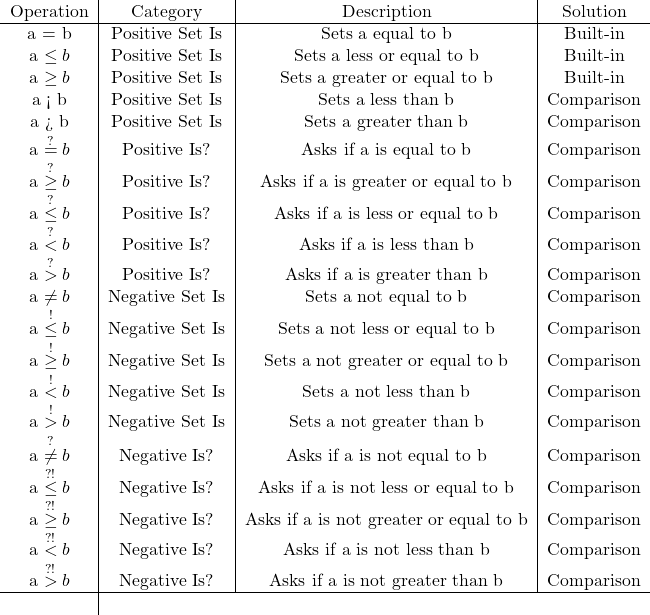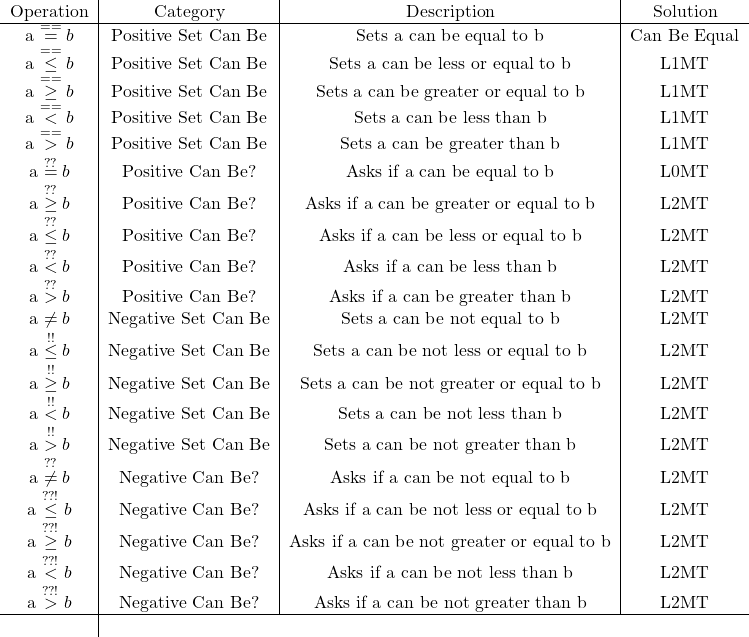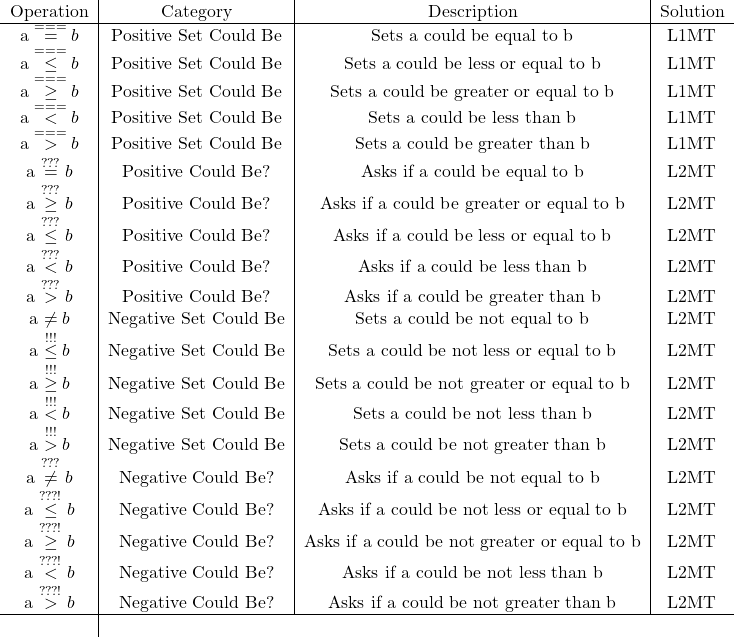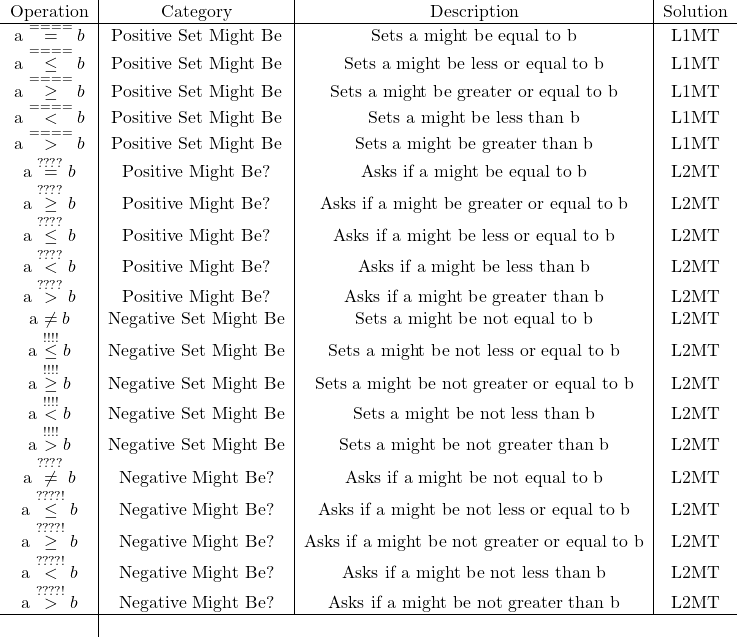# ILP Part 79 — Complex constraints and comparisons

This is the seventieth ninth part of the ILP series. For your convenience you can find other parts in the table of contents in Part 1 – Boolean algebra

Today we are going to explore constraints and comparisons a little more. We already know how to compare integers, we sort of know how to do the same for real numbers (and we remember it’s dangerous). We’ll now try asking questions “what if”.

# Is

This part describes constraints we already covered. They should be easy to grasp.

## Set Is

We start with “set is” constraints which are directly built into the model. They include the following:

• Set Is Equal –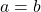• Set Is Less Or Equal –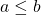• Set Is Greater Or Equal –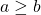Notice that these are “positive” constraints as they want to make “something to happen”. Each solver supports them.

## Positive Is?

• Is Equal –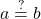• Is Greater Or Equal –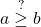• Is Less Or Equal –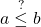• Is Greater Than –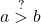• Is Less Than –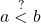We can calculate all of them by using regular comparisons we already defined.

## Positive Set Is

Rest of “positive is” constraints we can implement by using comparisons:

• Set Is Greater Than –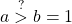• Set Is Less Than –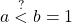## Negative Is?

Now we enter the world of negative constraints. We may ask the following:

• Is Not Equal – equivalent to Is Greater Than or Is Less Than
• Is Not Greater or Equal – equivalent to Is Less Than
• Is Not Less Or Equal – equivalent to Is Greater Than
• Is Not Greater Than – equivalent to Is Less Or Equal
• Is Not Less Than – equivalent to Is Greater or Or Equal

Still, no magic in here. We just use Positive Is formulas.

## Negative Set Is

Finally, we may want to add constraints that something doesn’t happen. They are obvious as well, we just take Negative Is and set them to true.

# Can be

Okay, now things get a little trickier. Previously we were asking whether something “is” happening. Now we ask if something “can be” happening. How is this useful? Let’s take this problem with integer variables: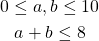with goal to minimize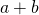. The optimal solution is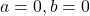as the sum is zero then.

## Positive Can Be?

We may ask the following about variable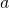whereis either variable or constant:

• Can Be Equal –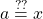• Can Be Greater Or Equal –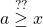• Can Be Less Or Equal –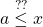• Can Be Greater Than –• Can Be Less Than –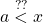What do they do? They answer whethercan be changed (but does not need to) in the final solution to particular option in a way that the model is still feasible, no other variable gets changed, and the goal function is allowed to change. So we may have the following examples:

•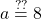– true as if we set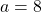then model is still feasible
•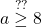– true based on the same reasoning
•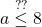– true as it’s already met
•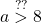– false as thenwould be too big
•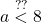– true

Notice however that if we set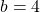then we get the following answers:

•– false as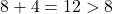•– false based on the same reasoning
•– true as we can set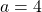•– false as thenwould be too big
•– true

So notice that we didn’t modifyin any way, we only modified some other variable and still we affected. Now, how do we implement these constraints?

### Can Be Equal?

This is the simplest one and actually something we can implement. We just need to imagine thatis indeed equal to something and then replicate all constraints including it. This is called “Level 0 Model Transformation” (L0MT). So in our case it would be: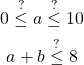And now we take conjunction of all these “positive is” constraints.

### Other Positive Can Be?

Other Positive Can Be constraints can’t be represented that easily. That’s because when we askit may be that it’s true for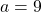but false for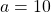so we don’t know which value to use. This can be represented using Level 2 Model Transformation (L2MT) explained later.

## Positive Set Can Be

Now we discuss constraints setting whether something can happen. We can implement them in some ways.

### Set Can Be Equal

This is the simplest one. Since we know what value we’re talking about, we can just substitute it as inand then make this true.

### Other Positive Set Can Be

For other constraints we need to use “Level 1 Model Transformation” (L1MT). We introduce a new variable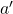and then check for constraints. Let’s see an example for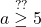. We start with this model: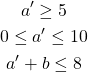We can see that solver needs to find an assignment meeting constraints. The model must be feasible so we don’t break anything.

## Negative Can Be?

• Can Be Not Equal – equivalent to Can Be Greater Than or Can Be Less Than
• Can Be Not Greater Or Equal – equivalent to Can Be Less Than
• Can Be Less Or Equal – equivalent to Can Be Greater Than
• Can Be Greater Than – equivalent to Can Be Less Or Equal
• Can Be Less Than – equivalent to Can Be Greater Or Equal

Notice that Can Be Not Greater Or Equal and Not Can Be Greater Or Equal are not equivalent anymore. The former effectively asks if there is a value Not Greater Or Equal to something which makes the model feasible, the latter negates the constraint which is different for infeasible models.

To implement these constraints we can’t introduce new variables as in case of negative answers the model would become infeasible. However, we can represent them using L2MT.

## Negative Set Can Be

And now we consider constraints. Again, they can be represented using L2MT as if we added some more variables and constrain them then we would end up with infeasible model.

# Could Be

Now we go similar route but allow to change some other variables as well (not all of them).

## Positive Could Be?

Let’s take this model: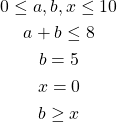is not very useful here but actually adds some constraint to depict the principle. Let’s say that we ask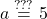so ifcould be equal to five if we are allowed to change some other variables. The answer is yes as we may setequal to five if we change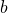. However,remains unchanged.

These constraints can be represented using L2MT. We cannot go with copying variables as for negative answer we would make model infeasible.

## Positive Set Could Be

While we cannot answer the question easily, we can make the constraint. We go with L1MT, copy variables and then add constraints. We would need to make sure that only some variables change which adds a little bit of complexity.

## Negative Could Be? and Negative Set Could Be

Again, we need to remember that Could Not Be Equal and Not Could Be Equal are not equivalent in terms of infeasibility. We can represent these by using L2MT.

# Might Be

Now we enter the world when all variables can change values.

## Positive Might Be? and Negative Might Be?

For these we basically ask if there is any solution with constraint met. Again, copying variables is not helpful. We need to go with L2MT.

## Positive Set Might Be and Negative Set Might Be

Now, these are very interesting. In the former we need to guarantee that there exists any solution which can be done with L1MT. For the latter we need to make sure that no solution exists which requires L2MT. However, notice that in the latter case we’re very close to the halting problem so in some cases it may not be possible at all.

# Model Transformations

Okay, but what are these all model transformations?

## Level 0 Model Transformation

In this transformation we already know all the values as we modify only one variable to some constant so we don’t need to introduce any other free-ish variables. What we need is to verify if all other constraints hold which we can do by replacing them with Positive Is? and Set Positive Is.

## Level 1 Model Transformation

In this case we need to introduce free-ish variables and include them in the model we solve. This is somewhat similar to Type 1 Hypervisor in VMs. We sort of build a model on the side and solve it when solving the original model.

## Level 2 Model Transformation

Now something interesting happens. When we need to make sure something is not possible then we need to create a model where something is impossible. But if we included that model in the original one then we would make the latter infeasible as well. So how do we do it?

The answer is Turing Machine. We know we can emulate Turing Machine in ILP and so we can emulate the ILP solving algorithm inside the model. Since we know how big the input is, we know the complexity, we can calculate the number of steps required to land at solution. So we effectively emulate a computer inside our model to solve some other model.

This is like Level 2 Hypervisors which need to do some emulation. We effectively do the same here. Obviously, it’s only theoretically possible now (as it would lead to gigantic number of variables) but can be represented in ILP.

# Summary

Now all operations at a glance: